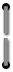# Appendix C

### Computing Time to Maturity

In computing time to maturity, the 31st of a month is always considered the same as the first of the following month. For example, if the settlement date is 11/15/72 and maturity is on 1/1/81, the time to maturity is 8 years, 1 month and 15 days.
Accrued interest on bonds is to be added to principal—computed for elapsed months and days on a 360-day year basis. In this computation a month is considered to be 1/12th of 360 days, or 30 days, and each period from a date in one month to the same date in the following month shall be considered to be 30 days. In the calculation, the first day of the accrual period should be counted, and the last or settlement date omitted.60